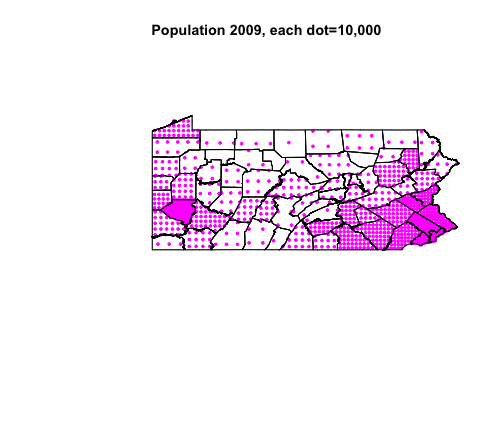Maptools

author: Chris Inkpen
date: February 2014

R maptools tutorial - Penn State R User Group
Adapted from a tutorial from University of Oregon Geography
http://www.geog.uoregon.edu/GeogR/examples/maps_examples01.htm

Exploratory Spatial Data Analysis

“Exploratory spatial data analysis is a set of techniques to describe and visualize spatial distributions, identify atypical locations or spatial outliers, discover patterns of sptial association, clusters or hot spots, and suggest spatial regimes or other forms of spatial heterogeneity (Dell'arba, 2005: Anselin, 1988).”

Maptools has a number of functions to explore the spatial relations in your data.

Set Working Directory

setwd("/Users/Chris/Desktop/Big Data stuff/R Resources/R scripts/Maptools/Rmaptools/subRmaptools")

This is going to be the folder where you have stored your PA shape files.

Install Packages

The 2nd step is to install the packages you will need for this tutorial.

# install.packages('rgeos') install.packages('maptools')
# install.packages('RColorBrewer') install.packages('classInt')

library(maptools)  #loads sp library and maptools package
## Checking rgeos availability: TRUE
library(RColorBrewer)  #for color scheme creation
library(classInt)  #assigns class intervals of continuous variables
library(rgeos)  #library for polygon clipping
## rgeos version: 0.3-3, (SVN revision 437)
##  GEOS runtime version: 3.3.3-CAPI-1.7.4
##  Polygon checking: TRUE

3rd step is to load in the PA county line files, the substantive point data (hospital data in this case), and the PA county shape files.

Exploring our data

For the 4th step, we'll take a look at our data.

Use the summary() function to get a better idea of what each layer looks like and see the structure and contents of the files.

# summary(pacounty) summary (Hospitalsc) summary(papop2)

Attributes

We'll use the attributes() function to get an idea of types of characteristics in each layer. You can remove the @ to see a complete list of observations.

# attributes(pacounty@data) attributes(papop2@data)

Mapping

R's maptools package can plot simple maps that read and plot ESRI shapefiles and display selected variables.

Map 1 - Map 1 plots the 2009 Population density by county in equal frequency class intervals. We will break this up into 2 blocks of code to see how R takes the necessary ingredients for a choropleth and then “bakes” them into various layers.

1st Block of Code - the ingredients.

# 1st Block of Code - the ingredients.
plotvar <- papop2@data\$PAPOP2_c_1
nclr <- 8
plotclr <- brewer.pal(nclr, "Oranges")
class <- classIntervals(plotvar, nclr, style = "quantile")
colcode <- findColours(class, plotclr)

2nd Block of code - plotting the layers.

plot(pacounty, xlim = c(-82, -74.7), ylim = c(38, 43))
plot(papop2, col = colcode, add = T)
title(main = "Pennsylvania Population in 2009", sub = "Quantile (Equal-Frequency) Class Intervals")
legend(-82, 39.5, legend = names(attr(colcode, "table")), fill = attr(colcode,
"palette"), cex = 0.6, bty = "n")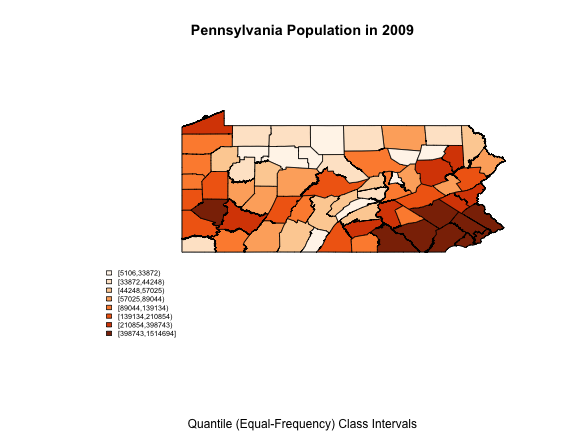The 2nd block of code produces the choropleth. The first “plot()” function sets up the map with specific long and lat window and plots the polygons. The second “plot()” function plots those polygons but with the assigned color code. The legend() function puts the legend in and the first two arguments (-82, 39.5) tells R where to put the legend (in long and lat)

2nd map - This map plots the number of licensed MDs per hospital from the hospital csv file and the basemap. This plots the hospitals where they actually are on the map (using lat/long coordinates).

1st block - ingredients

plotvar <- Hospitalsc\$Lic_MDs  # This takes the hospitals csv file and  specifies the column titled 'Lic_MDs' that stands for the number of licensed medical doctors in a particular hospital.
nclr <- 8
plotclr <- brewer.pal(nclr, "Blues")
class <- classIntervals(plotvar, nclr, style = "quantile")
colcode <- findColours(class, plotclr)

2nd block - plotting layers

plot(pacounty, xlim = c(-82, -74.7), ylim = c(38, 43))  # This plots the map.
points(Hospitalsc\$X, Hospitalsc\$Y, pch = 16, col = colcode, cex = 1)  # THis plots points of # of licensed mds on the specific lat/long points using the 'points()' function.
points(Hospitalsc\$X, Hospitalsc\$Y, cex = 1)  # this puts a black circle around each point to help distinguish plots.
title(main = "Licensed MDs per Hospital in PA", sub = "Quantile (Equal-Frequency) Class Intervals")
legend(-82, 39.5, legend = names(attr(colcode, "table")), fill = attr(colcode,
"palette"), cex = 0.6, bty = "n")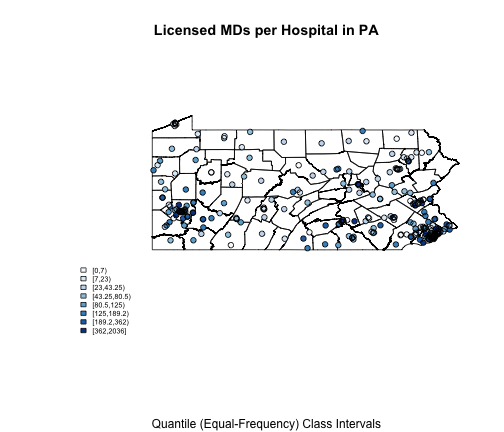3rd map - plotting both county population density and licensed MDs per Hospital.

In this exercise, we'll actually have 3 blocks of code. The first will be the ingredients for the PA population density by county, the second will be the point data for the licensed MDs per hospitals, and the third will be the plot of all the layers.

# 1st Block of Code - the ingredients for population
plotvar <- papop2@data\$PAPOP2_c_1  # This creates an object of what we want to plot. In this case population.
nclr <- 8  # This tells R the number of class intervals to use.
plotclr <- brewer.pal(nclr, "Oranges")  # defines the plot color package.
class <- classIntervals(plotvar, nclr, style = "quantile")  # what type of class interval. In this case, quantiles.
colcode <- findColours(class, plotclr)  # This assigns the colors.

# 2nd Block of code - ingredients for licensed MDs per hospital
plottry <- Hospitalsc\$Lic_MDs  # This takes the hospitals csv file and  specifies the column titled 'Lic_MDs' that stands for the number of licensed medical doctors in a particular hospital.
nclr2 <- 8
plotclr2 <- brewer.pal(nclr, "Blues")
class2 <- classIntervals(plottry, nclr2, style = "quantile")
colcode2 <- findColours(class2, plotclr2)

# 3rd block - plotting layers
plot(pacounty, xlim = c(-82, -74.7), ylim = c(38, 43))  # This plots the map.
plot(papop2, col = colcode, add = T)  # This plots the assigned color code layer.
points(Hospitalsc\$X, Hospitalsc\$Y, pch = 16, col = colcode2, cex = 1)  # THis plots points of # of licensed mds on the specific lat/long points using the 'points()' function.
points(Hospitalsc\$X, Hospitalsc\$Y, cex = 1)  # this puts a black circle around each point to help distinguish plots.
title(main = "Licensed MDs per Hospital in PA by Population Density", sub = "Quantile (Equal-Frequency) Class Intervals")
legend(-82, 39.5, legend = names(attr(colcode, "table")), fill = attr(colcode,
"palette"), cex = 0.6, bty = "n")
legend(-79, 39.5, legend = names(attr(colcode2, "table")), fill = attr(colcode2,
"palette"), cex = 0.6, bty = "n")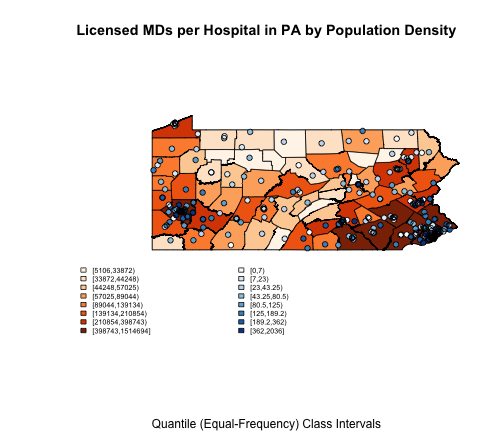Section 2: Cartograms and Dot-Density Maps

Despite legends and color schemes, area in maps can still act to mislead viewers, making larger areas seem more important even if they possess a lower amount of the variable of interest.

One method to get around this is to plot a cartogram; a bubble plot that places circles of varying size depending on the amount of the variable of interest over the polygon (in this case, the county).

bubble plot cartograms

plotvar <- papop2@data\$PAPOP2_c_1
nclr <- 8
plotclr <- brewer.pal(nclr, "BuPu")
# plotclr <- plotclr[nclr:1] # reorder colors if appropriate
max.symbol.size = 4  # the thing that says biggest point is size 4
min.symbol.size = 1  # the thing that says the smallest point is point 1
class <- classIntervals(plotvar, nclr, style = "quantile")
colcode <- findColours(class, plotclr)
symbol.size <- ((plotvar - min(plotvar))/(max(plotvar) - min(plotvar)) * (max.symbol.size -
min.symbol.size) + min.symbol.size)  # This is the part that sets the symbol size, it takes the value for one observation on pop'n1900, substracts the minimum of that whole variable. Divides this by the max of the variable minus the min multiplied by the max.symbol.size minus the min.symbol.size.
plot(papop2, xlim = c(-82, -74.7), ylim = c(38, 43))
papop2.cntr <- coordinates(papop2)
points(papop2.cntr, pch = 16, col = colcode, cex = symbol.size)  # setting the change expression to the 'symbol.size' variable
points(papop2.cntr, cex = symbol.size)  #puts a black ring on the points.
title(main = "Cartogram of PA Counties by 2009 Population Density", sub = "Equal-Interval Class Intervals")
legend(-79, 39.5, , legend = names(attr(colcode, "table")), fill = attr(colcode,
"palette"), cex = 0.6, bty = "n")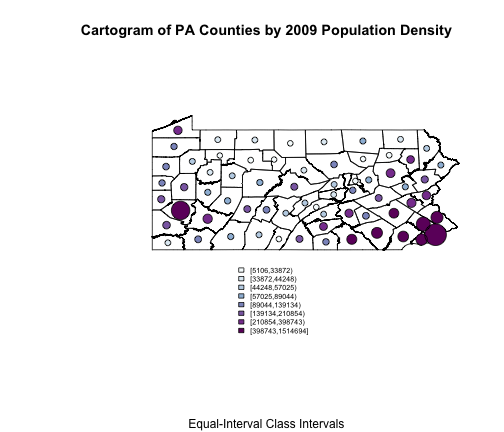Here the size of each symbol is calculated using the county pop'n and the code at the end of the 1st block. The symbol is plotted at the centroid of each county, which is located using the coordinates() function.This must just take the centroid of each polygon in the shape file.

Dot-density maps

This first map plots random location dots (within the counties) to represent the population in ten thousands. It is important to divide the variable of interest by some large'ish number because otherwise it will take a very long time to plot out all the points within each county.

plottitle <- "Population 2009, each dot=10,000"
papolys <- SpatialPolygonsDataFrame(papop2, data = as.data.frame(papop2))
plotvar <- papolys@data\$PAPOP2_c_1/10000

1st map - random dot-density

dots.rand <- dotsInPolys(papolys, as.integer(plotvar), f = "random")
plot(papolys, xlim = c(-82, -74.7), ylim = c(38, 43))
plot(dots.rand, add = T, pch = 19, cex = 0.5, col = "blue")
title(plottitle)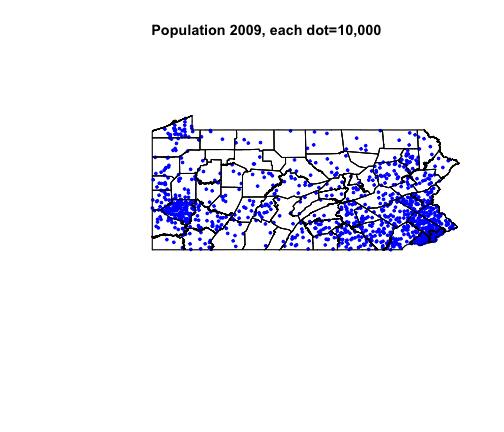2nd map - regular dot-density (wallpaper style)

dots.reg <- dotsInPolys(papolys, as.integer(plotvar), f = "regular")
plot(papolys, xlim = c(-82, -74.7), ylim = c(38, 43))
plot(dots.reg, add = T, pch = 19, cex = 0.5, col = "magenta")